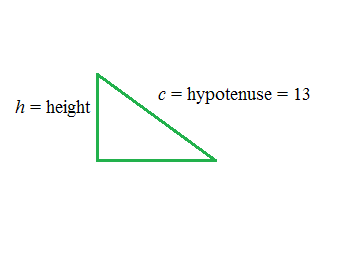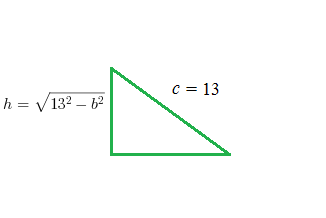### Home > CALC3RD > Chapter Ch7 > Lesson 7.1.3 > Problem7-32

7-32.

A right triangle has a fixed hypotenuse of length $13$ units. If a leg is increasing in length at a rate of $\frac { 1 } { 2 }$ unit per second, find the rate of change in area of the triangle when that same leg is $5$ units long.

Sketch the triangle and label the known information.

So start with an area equation: $A=\frac{1}{2}bh$.

Notice that there are two variables. It would be easier to have only one.

Use the Pythagorean Theorem to find the length of the height in terms of $b$.
Then rewrite the function with only one variable:

$A=\frac{1}{2}(b)(\sqrt{13^{2}-b^{2}})$Implicitly differentiate with respect to time to find a function for $\frac{dA}{dt}$,

the rate that the area is changing.

Substitute in all values that you know, including the specific base length, $5$.

If you did it right, the only variable that will remain is $\frac{dA}{dt}$.